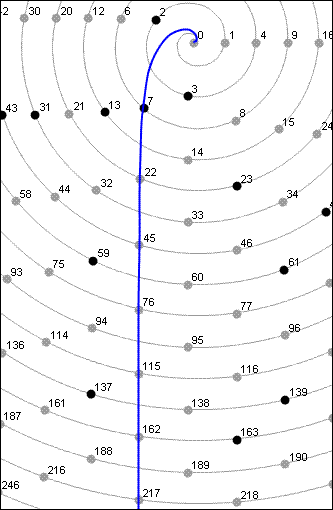NumberSpiral.com

CONTENTS6.  Composite offset curves

 On a previous page we learned that the quadratic formula for composite offset curves is: y = ax2 + bx Such numbers can be factored like this: y = x(ax+b) This factorization explains why all the integers on these curves are composite except for the first (where x is zero) and possibly the second (where x is one). As an example, let's look at the first composite offset curve of angle 3/4. From the information on this page, we know that the coefficients of its corresponding quadratic function are a = 4 b = 3 Therefore the function is y = 4x2 + 3x which tells us that the curve's integers can be factored like so: x(4x+3) This allows us to make the following table of the integers on the curve:

 Index Factorization Integer 0 0 x 3 0 1 1 x 7 7 2 2 x 11 22 3 3 x 15 45 4 4 x 19 76 5 5 x 23 115 6 6 x 27 162 7 7 x 31 217For comparison, here's a screenshot of the same numbers on the spiral:VortexTo draw this curve with Vortex, enter "coe 4 3 0".

Figure 11  2  3  4  5  6  7  8  9  10   Next